# 4.4. pyopus.optimizer.coordinate — Box constrained coordinate search optimizer¶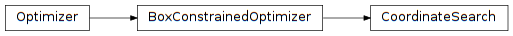Box constrained coordinate search optimizer (PyOPUS subsystem name: CSOPT)

The algorithm (asymptotically) converges to a point where the projection of the cost function gradient is normal to the active constraint (i.e. stationary point in the sense of constrained optimization) if the following holds

• the cost function is continuously differentiable,
• the step size is a rational multiple of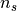(see BoxConstrainedOptimizer),
• the step scaling factors stepup and stepdn are rational.

If the above conditions hold the algorithm can be classified as a pattern search algorithm. For a proof see

Lewis R. M., Torczon V.: Pattern search algorithms for bound constrained minimization. SIAM Journal on Optimization, vol. 9, pp. 1082-1099, 1999.

class pyopus.optimizer.coordinate.CoordinateSearch(function, xlo=None, xhi=None, debug=0, fstop=None, maxiter=None, stepup=1.0, stepdn=0.5, step0=None, minstep=None)

Coordinate search optimizer class

stepup is the factor by which the step size is increased after a successfull step.

stepdn is the factor by which the step size is decreased after steps in all directions fail.

step0 is the initial vector of ndim step sizes corresponding to coordinate directions. It can also be a scalar in which case it is applied to all directions.

minstep is a vector of step sizes used in the stopping condition. After all step sizes fall below the corresponding values in minstep the algorithm is stopped. minstep can also be a scalar in which case it applies to step sizes in all directions.

If step0 and minstep are not given they are set to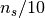and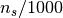when the reset() method is called.

Box constraints are handled by setting the components of a vector that violate a bound to the bound.

Infinite bounds are allowed. This means that the algorithm behaves as an unconstrained algorithm if lower bounds are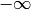and upper bounds are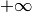.

See the BoxConstrainedOptimizer class for more information.

check()

Checks the optimization algorithm’s settings and raises an exception if something is wrong.

reset(x0)

Puts the optimizer in its initial state and sets the initial point to be the 1-dimensional array x0. The length of the array becomes the dimension of the optimization problem (ndim member). The shape of x must match that of xlo and xhi.

run()

Runs the optimization algorithm.

#### Previous topic

4.3. pyopus.optimizer.filter — Filter-based point acceptance

#### Next topic

4.5. pyopus.optimizer.hj — Box constrained Hooke-Jeeves optimizer